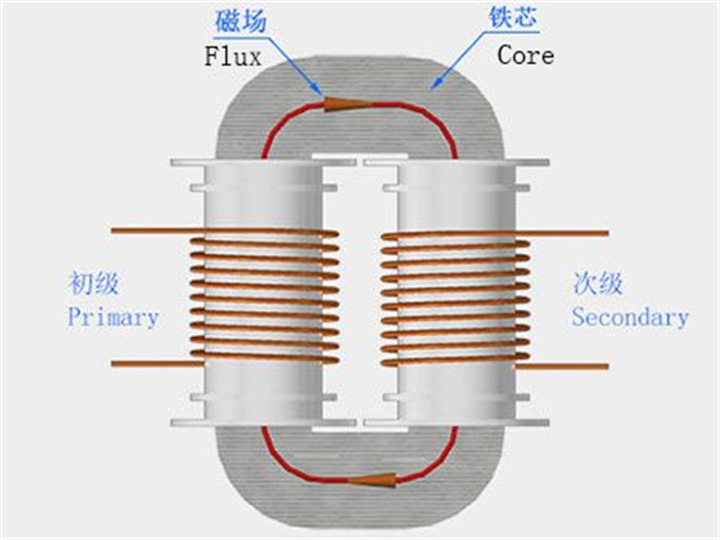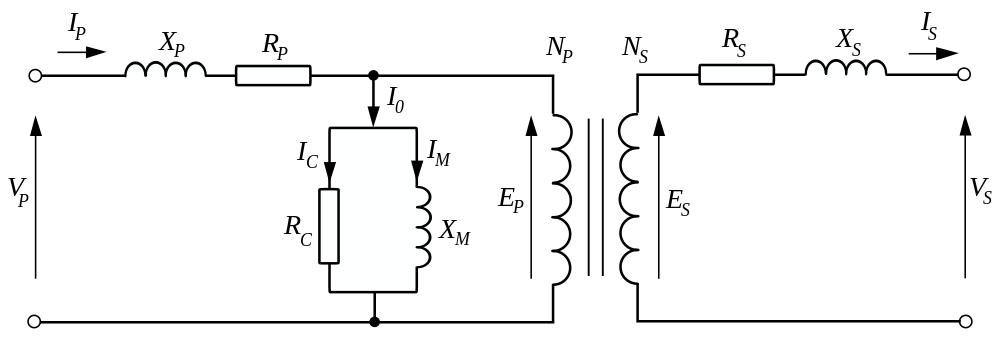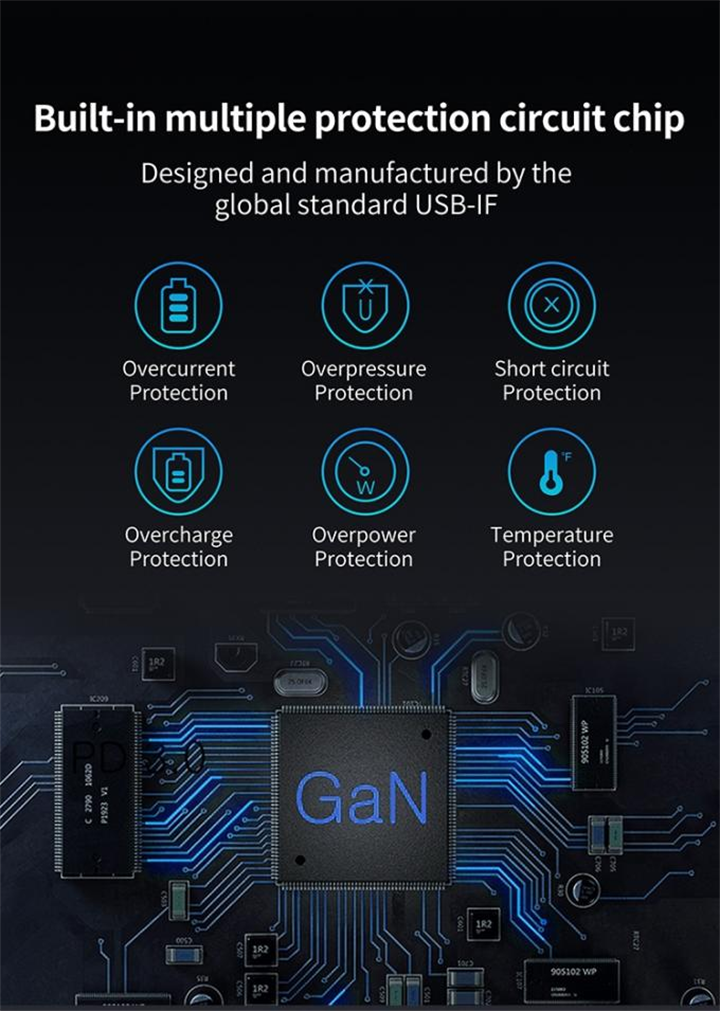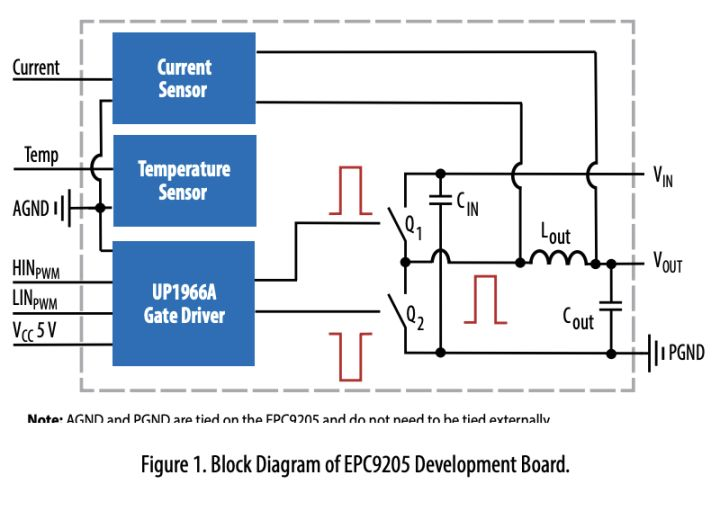# Why are gallium nitride chargers so good?Just remember one thing. It is a very traditional transformer.In fact, as shown in the figure above, the ratio between input voltage and output voltage is equal to the ratio of the number of coils (input voltage/output voltage=number of input coils/number of output coils). This is a perfect electromagnetic=>magnetoelectric conversion. There is also a formula: input current x number of input coils=output current x number of output coils. In this way, the voltage and current of the power supply can be controlled. The general basic structure circuit diagram is as followsSo why is the GaN charger so powerful?Gallium nitride is mainly used to increase frequency. In a transformer, the higher the frequency, the more energy that can be converted through electromagnetic effects. Therefore, the switching circuit made of gallium nitride can greatly improve the frequency of the AC circuit of the transformer. In this way, the silicon MOSFET is slowly converted to gallium nitride.

In fact, the circuit of the above separated parts is not very common now. Basically, they are processed by the integration block. It is usually a power device that is used as the master. The power converter is like this. The converted power is proportional to the volume. Therefore, the chargers made of materials in the past can not be smaller to achieve a certain power. Gallium nitride is the relationship between power and volume, which can be minimized. That is to say, the power may be very high, but I can make the volume very small because of my own materials. At the same time, the circuit is quite simple.

This is the basic logic circuit. There is nothing wrong with this thing.As a result, modern chargers are getting smaller and smaller, and their power is getting higher and higher.

The principle of gallium nitride is not correct. In fact, the main function of gallium nitride is to turn 220V 50Hz mains power into high-frequency current through its own switching circuit. The original silicon MOS tube is Apple’s technology generation, and the switching frequency can be about 50000 times. After using gallium nitride, the switching frequency can reach millions. In this way, the overall charging power is higher than that of Apple, and the volume has not changed. While maintaining this high power, there is also a certain amount of low heat because of the recovery technology, which uses two gallium nitride to do the same thing. But in this respect, Oppo is the best in terms of volume, because it has customized a set of control devices and used some small ceramic capacitors. Therefore, the overall volume is smaller, and the charging power can be guaranteed while maintaining good heat generation.

Post time: Dec-14-2022